# Convection equations

Consider a fluid flow in a layer of uniform depth where the temperature difference,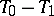, between the upper and lower bounding planes is kept constant. Such a system has a steady-state solution in which there is no fluid motion and the temperature varies linearly. If this solution is unstable, convection should develop. When all motion is parallel to the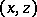-plane, the governing equations are [a1]: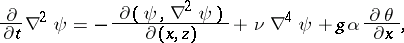whereis the height of the layer (in the-direction),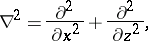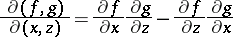stands for the Jacobian determinant,is a stream function for the two-dimensional fluid motion, andis the deviation of the temperature from the case where no convection occurs. The coefficients,,,are, respectively, kinematic viscosity, gravity acceleration, thermal expansion, and thermal conductivity. The part of the first equation that does not depend uponis the third component of the vorticity equation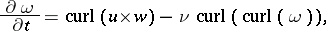whereis the velocity vector andis the vorticity.

See [a4], [a5], and also Curl and Vector product.

By expandingandin double Fourier series with coefficients depending onand truncating to three terms, the Lorenz equations result [a2].